# Introduction

Review of basic algebraic terms:

 Algebraic term Description Example
 Algebraic expression A mathematical phrase that contains numbers, variables (letters), and arithmetic operations (+, – , ×, ÷, etc.). 3x – 4 5a2 – b + 3 12y3 + 7y2 – 5y +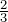Constant A number on its own. 2y + 5        constant:        5 Coefficient The number in front of a variable. -9x2    coefficient:    -9  x    coefficient:    1 (x = 1 · x) Term A term can be a constant, a variable, or the product of a number and variable. (Terms are separated by a plus or minus sign.) 2x3 + 7x2 – 9y – 8 Terms: 2x3,   7x2,   – 9y,   –8 Like terms The terms that have the same variables and exponents (differ only in their coefficients). 2x      and     -7x -4y2      and      9y2 0.5pq2      andpq2

Polynomial: an algebraic expression that contains one or more terms.

Example:    7x ,       5ax – 9b ,       6x2 5x +,       7a2 + 8b + ab – 5

There are special names for polynomials that have one, two, or three terms:

• Monomial: an algebraic expression that contains only one term.

Example:       9x ,       4xy2 ,       0.8mn2  ,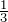a2b

• Binomial: an algebraic expression that contains two terms.

Example:          7x + 9 ,        9t2 – 2t ,         0.3y +• Trinomial: an algebraic expression that contains three terms.

Example:          ax2 + bx + c ,       4qp2 + 3q + 5

# Combining Terms

Like terms: terms that have the same variables and exponents (the coefficients can be different).

Examples:

 Example Like or unlike terms
 7y   and   -9y Like terms 6a2,   -32a2,   and   –a2 Like terms 0.3 x2y   and   -48x2y Like terms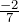u2v3   and   u2v3 Like terms -8y   and   78x Unlike terms 6m3   and   -9m2 Unlike terms -9u3w2   and   -9w3u2 Unlike terms

Combine like terms: add or subtract their coefficients and keep the same variables and exponents.

Note: unlike terms cannot be combined.

Example 1.1.1

 1) 3a + 7b – 9a + 15b = (3a – 9a) + (7b + 15b) Regroup like terms. = -6a + 22b Combine like terms.
 2) 2y2 – 4x + 3x – 5y2 = (2y2 – 5y2) + (-4x + 3x) Regroup like terms. = -3y2 – 1x Combine like terms. = -3y2 – x
 3) 8xy2 – x2y + 4x2y – 6xy2 = 8xy2 – x2y + 4x2y – 6xy2 Or underline like terms without regrouping. = 2xy2 + 3x2y Combine like terms.
 4) 2(2m + 3n) + 3(m – 4n) = 4m + 6n + 3m – 12n Distributive property. = 7m – 6n Combine like terms.

# Removing Parentheses

If the sign preceding the parentheses is positive (+), do not change the sign of terms inside the parentheses, just remove the parentheses.

Example:     (x 5) = x 5

If the sign preceding the parentheses is negative (-), remove the parentheses and the negative sign (in front of parentheses), and change the sign of each term inside the parentheses.

Example:    – (x 7) =x + 7

Remove parentheses:

 Algebraic expression Remove parentheses Example
 (ax + b) ax + b (5x + 2) = 5x + 2 (ax – b) ax – b (9y – 4) = 9y – 4 – (ax + b) -ax – b – (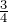x + 7) = –x – 7 – (ax – b) -ax + b – (0.5b – 2.4) = -0.5b + 2.4

Example 1.1.2

 1)  9x2 + 7 – (2x2 – 2) = 9x2 + 7 – 2x2 + 2 Remove parentheses. = 7x2 + 9 Combine like terms.
 2)  (-8y + 5z) – 4(y – 7z) = -8y + 5z – 4y + 28z Remove parentheses. = -12y + 33z Combine like terms.
 3)   – (3a2 + 4a – 4) + 3(4a2 – 6a + 7) Remove parentheses. = – 3a2 – 4a + 4 + 12a2 – 18a + 21 Distributive property. = 9a2 – 22a + 25 Combine like terms.
 4)   -5(u2 – 3u) + 3(2u – 4) – (5 – 3u + 4u2) Distributive property. = -5u2 + 15u + 6u – 12 – 5 + 3u – 4u2 Remove parentheses. = -9u2 + 24u – 17 Combine like terms.

# Multiplying and Dividing Algebraic Expressions

Multiplying a monomial and a polynomial:

• Use the distributive property: a (b + c) = ab + ac
• Multiply coefficients and add exponents with the same base. Apply am an = am+n

Example 1.1.3

 1) 3x3 (5x2 – 2x) = (3x3) (5x2) – (3x3) (2x) Distributive property:  a (b + c) = ab + ac = (3 ∙ 5) (x3 x2) – (3 ∙ 2) (x3 x1) Regroup           x = x1 = 15 (x3+2) – 6 (x3+1) Multiply the coefficients & add the exponents. = 15x5 – 6x4 am ∙ an = am+n
 2) 5ab2 (2a2b +ab2 – a) Distribute. = (5ab2) (2a2b) + (5ab2) (ab2) + (5ab2) (-a) Multiply the coefficients and add exponents. = (5 ∙ 2) (a1+2 b2+1) + (5a1+1 b2+2) – (5a1+1b2) b = b1     ,     a = a1 = 10a3b3 + 5a2b4 – 5a2b2 am ∙ an = am+n

Dividing a polynomial by a monomial:

• Split the polynomial into several parts.
• Divide a monomial by a monomial. Apply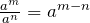.

Example 1.1.4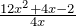Steps                                                               Solution

• Split the polynomial into three parts: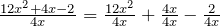• Divide a monomial by a monomial: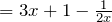# The FOIL method: an easy way to find the product of two binomials (two terms).

 (a + b) (c + d) = ac + ad + bc + bd                            F      O      I      L Example
 F – First terms first term × first term               (a + b) (c + d) (x + 5) (x + 4) O – Outer terms outside term × outside term     (a + b) (c + d) (x + 5) (x + 4) I – Inner terms inside term × inside term         (a + b) (c + d) (x + 5) (x + 4) L – Last terms last term × last term                 (a + b) (c + d) (x + 5) (x + 4)

 FOIL method Example
 (a + b) (c + d) = ac + ad + bc + bd (x + 5) (x + 4) = x ∙ x + x ∙ 4 + 5x + 5 ∙ 4 = x2 + 9x + 20 F     O      I       L F        O       I       L

Multiplying binomials (2 terms × 2 terms):

Example 1.1.5

 1)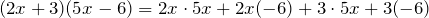F             O           I             L The FOIL method.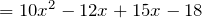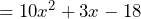Combine like terms.

 2)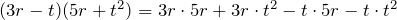FOIL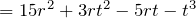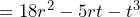Combine like terms.

 3)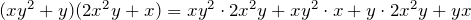FOIL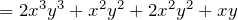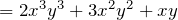Combine like terms.

 4)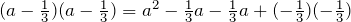FOIL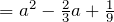Combine like terms.

# Practice questions

1. Identify the terms of each polynomial:

a. 5x3 + 8x2 + 2x

b.y4 + 9a2 + a – 1

2. Combine like terms:

a. 7x + 10y – 8x + 9y

b. 12a2  33b + 2b – 6a2

c. 13n + 5(6nm2) + 7(2m2 + 3n)

3. Simplify:

a. 15a2 + 9 – (5a2 – 4)

b. (-13x + 9y) – 6(x – 5y)

c. 5(ab – 2xy) – 6(-2ab + 3xy)

d. (5y – 7) (8y + 9)

e. (7r – 2t) (3r + 4t2)

f. (x) (x)